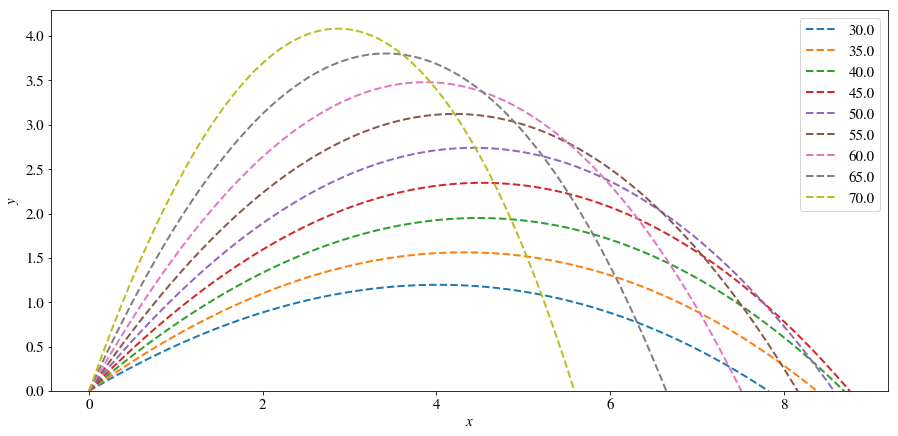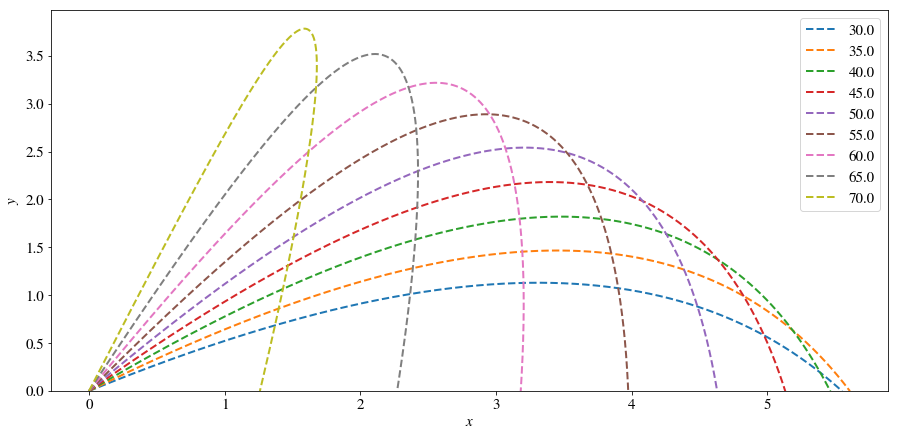# Throw into the Wind with Air Drag¶

### Examples - Mechanics¶

Last edited: March 15th 2018

In this example we will study the trajectory of a ball that is moving through the air, subject to air drag and wind. We expect the optimal angle to change compared to no drag and/or no wind, meaning the angle under which we can throw the ball the furthest for a given launch speed. Let us apply Newton’s law and solve the governing equations numerically. In particular, we launch a ball at the origin within the $xy$-plane at an angle $\theta$ with the horizontal and initial speed $v_0$.

### Governing Equations¶

Newton's second law states that $$\vec{F} = m\vec{a},$$ where $\vec{F}$ is the force acting on the ball, $\vec{a}$ is the acceleration, and $m$ is the mass of the ball. In this example there are two forces acting on the ball: the gravitational force $$m\vec{g}$$ and air drag $$-\frac{\rho}{2}C_DA\left|\vec{v}-\vec{V}\right|\left(\vec{v}-\vec{V}\right),$$ where $\rho$ is the air density, $A$ the ball’s projected area, $\vec{v}$ the velocity of the ball, $\vec{V}$ the wind speed, and $C_D\sim 0.47$ the friction coefficient.

This equation is only accurate for objects that move fast enough to generate turbulence. In this case the drag is proportional to the square of the relative velocity. A baseball thrown at over 80 mph is a good example. In contrast, for slow, non-turbulent motion $$− 6 \pi \eta R \left(\vec{v} − \vec{V} \right)$$ is a good model to describe the drag, where $\eta$ is the air’s viscosity and $R$ the ball’s radius. This is called Stokes’ law and can be found in most college-level textbooks on mechanical physics.

In a coordinate system where $x$ points horizontally along the ground and $y$ points vertically upwards, the governing equations become \begin{align} m\ddot{x} &= -\frac{\rho}{2}C_DA\left(\dot{x}-V_x\right)\left|\dot{\vec{r}}-\vec{V}\right|,\\ m\ddot{y} &= -\frac{\rho}{2}C_DA\left(\dot{y}-V_y\right)\left|\dot{\vec{r}}-\vec{V}\right|-mg, \end{align} where $V_x$ and $V_y$ are the $x$ and $y$ components of the wind velocity, and $$\left|\dot{\vec{r}}-\vec{V}\right| = \left[(\dot{x}-V_x)^2+(\dot{y}-V_y)^2\right]^{1/2}.$$

For simplicity, we assume that $V_y = 0$, i.e. the wind only blows horizontally. The one-dimensional governing equations are two second-order ordinary differential equations which can be written as four first-order differential equations in the following way: \begin{align} \dot{x} &= u,\\ \dot{u} &= -\frac{\rho}{2m}C_DA\left(u-V_x\right)\left[(u-V_x)^2+w^2\right]^{1/2},\\ \dot{y} &= w,\\ \dot{w} &= -\frac{\rho}{2m}C_DAw\left[(u-V_x)^2+w^2\right]^{1/2}-g \end{align}

### Discretization: Euler's Method¶

To solve the previous four equations numerically, we can discretize time: $$t \rightarrow t_n = t_0 + n\Delta t,$$ where $\Delta t$ is called the time step, and $n$ is the number of time steps. The derivative of $x$, for example, can then be approximated by $$\dot{x} \approx \frac{x_{n+1}-x_n}{\Delta t}.$$

Using Euler's method in this manner, the discretized version of the four first-order differential equations is \begin{align} x_{n+1} &= x_n + \Delta t \cdot u_n,\\ u_{n+1} &= u_n - \Delta t \frac{\rho}{2m}C_DA\left(u-V_x\right)\left[(u-V_x)^2+w^2\right]^{1/2},\\ y_{n+1} &= y_n + \Delta t \cdot w_n, \\ w_{n+1} &= w_n - \Delta t \left[\frac{g+\rho}{2m}C_DAw\left[(u-V_x)^2+w^2\right]^{1/2}\right], \end{align} where $x_n = x(t_n)$, $u_n = u(t_n)$ etc.

#### Initial Conditions¶

We now need to decide on the initial conditions, i.e. $x_0$, $y_0$, $u_0$ and $w_0$ at $t_0 = 0$. If we assume that the throw starts at the origin, we get $x_0 = y_0 = 0$. We further assume that the throw is made with velocity $v_0$ at an angle $\theta$, with $\theta = 0^\circ$ being a throw along the horizontal (positive $x$) axis and $\theta = 90^\circ$ being a throw straight up. This gives us $u_0 = v_0 \cos \theta$ and $w_0 = v_0 \sin \theta$.

### Implementation¶

First we import the relevant packages and set options for nicer plots.

In :
%matplotlib inline
import numpy as np
import matplotlib.pyplot as plt
from matplotlib import rc

newparams = {'figure.figsize': (15, 7), 'axes.grid': False,
'lines.markersize': 10, 'lines.linewidth': 2,
'font.size': 15, 'mathtext.fontset': 'stix',
'font.family': 'STIXGeneral'}
plt.rcParams.update(newparams)


The following code uses Euler's method to calculate and plot the trajectory of a throw in wind.

In :
def ThrowInWind(v0, theta0, windX, dt, t_max, collisionCheck, plotOn):
""" Function for simulation a throw in wind.

Parameters:
v0               # start velocity
theta0           # throw angle (degrees)
windX            # wind velocity in the x-direction
dt               # length of time step
t_max            # maximum simulation time
collisionCheck   # Bool, only simulates until ball hits ground if True
plotOn           # Bool, generates plots if True

Returns coordinates x and y.
"""

# Set values:
g = 9.81                # Gravity
m = 0.1                 # Mass of the ball
cd = 0.47               # Drag coefficient
rho = 1.15              # Air density in kg/m^3

area = np.pi*rad**2     # Projected area of the ball
k = rho*cd*area/(2*m)   # Air drag
n_max = int(t_max/dt)   # Number of time steps

x = np.zeros(n_max)
u = np.zeros(n_max)
y = np.zeros(n_max)
w = np.zeros(n_max)

theta0 = theta0*np.pi/180    # Convert throwing angle to radians
x = y = 0              # Define starting position
u = v0*np.cos(theta0)     # Initial velocity in x-direction
w = v0*np.sin(theta0)     # Initial velocity in y-direction

for n in range(n_max-1):
drag = k*dt*np.sqrt((u[n]-windX)**2 + w[n]**2)
x[n+1] = x[n] + dt*u[n]
u[n+1] = u[n] - drag*(u[n]-windX)
y[n+1] = y[n] + dt*w[n]
w[n+1] = w[n] - g*dt - drag*w[n]
if collisionCheck:
if y[n+1] <= 0:
x = x[0:n+2]
y = y[0:n+2]
break

if plotOn:
plt.plot(x,y)
plt.legend(["Ball trajectory"])
plt.ylabel(r"$y$")
plt.xlabel(r"$x$")
if collisionCheck:
plt.gca().set_ylim(bottom=0)
return x,y


#### Optimal Launch Angle¶

The following code can be used to estimate the optimal launch angle. The plot generated below is for when there is no wind.

In :
thetas = np.linspace(30, 70, 9)
for theta0 in thetas:
x, y = ThrowInWind(v0=10, theta0=theta0, windX=0, dt=0.001, t_max=3, collisionCheck=True, plotOn=False)
plt.plot(x,y,'--')

plt.legend(thetas)
plt.ylabel(r"$y$")
plt.xlabel(r"$x$")
plt.gca().set_ylim(bottom=0);We see that the optimal angle is approximately $45^\circ$. How does this angle change when there is wind, especially head wind?

In :
thetas = np.linspace(30, 70, 9)
for theta0 in thetas:
x, y = ThrowInWind(v0=10, theta0=theta0, windX=-10, dt=0.001, t_max=3, collisionCheck=True, plotOn=False)
plt.plot(x,y,'--')

plt.legend(thetas)
plt.ylabel(r"$y$")
plt.xlabel(r"$x$")
plt.gca().set_ylim(bottom=0);We see that with a head wind with velocity $10$, the optimal angle is lower than $45^\circ$, approximately $35^\circ$.

### Questions¶

• What role does the Time Step parameter play? What happens when this is set to high?
• Set the Simulation Time to 60 s. Can you explain why the ball’s path becomes linear? (hint: terminal velocity)
• Is it possible to make the ball do a loop-the-loop (a path that crosses itself)?